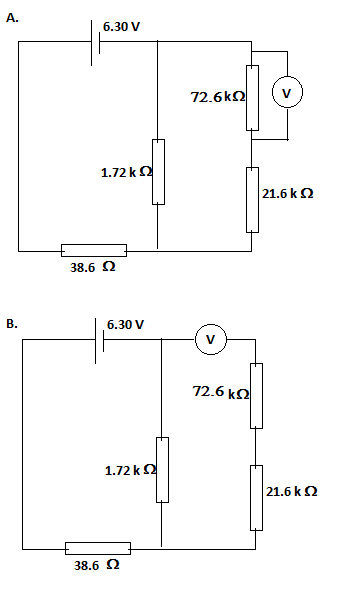a. Choose the circuit showing how a voltmeter would be connected to measure the voltage across...

Question:

a. Choose the circuit showing how a voltmeter would be connected to measure the voltage across {eq}72.6 \space k \Omega {/eq} resistor.

b. Assuming the voltmeter to be ideal, what is its reading?Voltmeter:

Like in most measuring instruments, the design considerations of the voltmeter are linked to the application. For example, the high internal resistance of the voltmeter, meant to minimize channeling circuit current to the meter and thus compromising the circuit parameters, also means that we connect the meter in parallel.

Become a Study.com member to unlock this answer! Create your account

Part (a)

{eq}\boxed{A.} {/eq}

A voltameter is connected across the two points of which the potential difference is required.

Part (b)

We...Parallel Circuits: Calculating Currents with Ohm's Law

from

Chapter 9 / Lesson 6
2.6K

Understand Kirchhoff's law and Ohm's law for parallel circuits, and see how to find current in a parallel circuit using the parallel circuit current formula.